# Illustrations of Support Vector Machines

##### Posted on May 18, 20170 Comments
Tags: SVM, ROC, Cross-Validation

Use the e1071 library in R to demonstrate the support vector classifier and the SVM.

## Implement in R

library(e1071)
svm() ## kernel = "linear"
## small "cost" means wide margins, while large "cost" means narrow margins


An intuition is that large “cost” $C$ would be more strict for the misclassification, and hence narrow margins. Specially, $C = \infty$ corresponds to the separable case.

Refer to 12.2 支持向量分类器 - ESL CN for more details.

## Support Vector Classifier

demonstration for two-dimensional example

### generate the data

set.seed(1)
x = matrix(rnorm(20*2), ncol = 2)
y = c(rep(-1 ,10), rep(1 ,10) )
x[y == 1, ] = x[y == 1, ] + 1
plot(x , col = 3-y )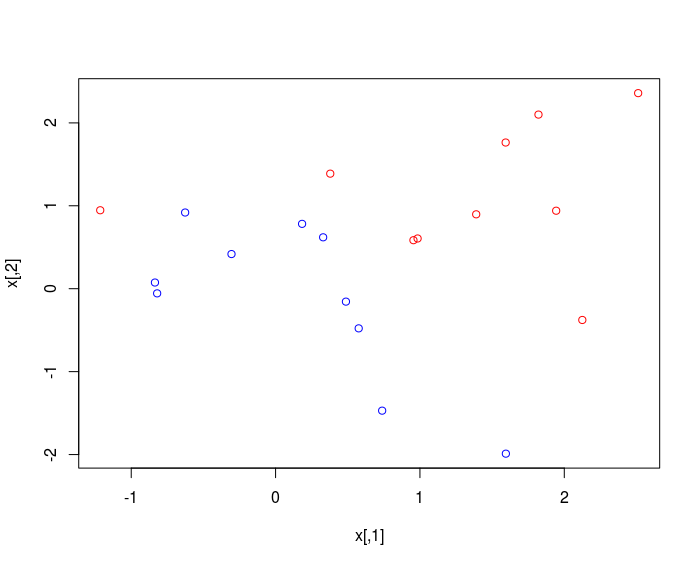The classes are NOT linearly separable.

### fit the support vector classification

It is worth noting that we must encode the response as a factor variable in order to perform classification.

dat = data.frame(x = x, y = as.factor(y))
library(e1071)
svmfit = svm (y ~ ., data = dat, kernel = "linear", cost = 10, scale = FALSE)
plot(svmfit, dat)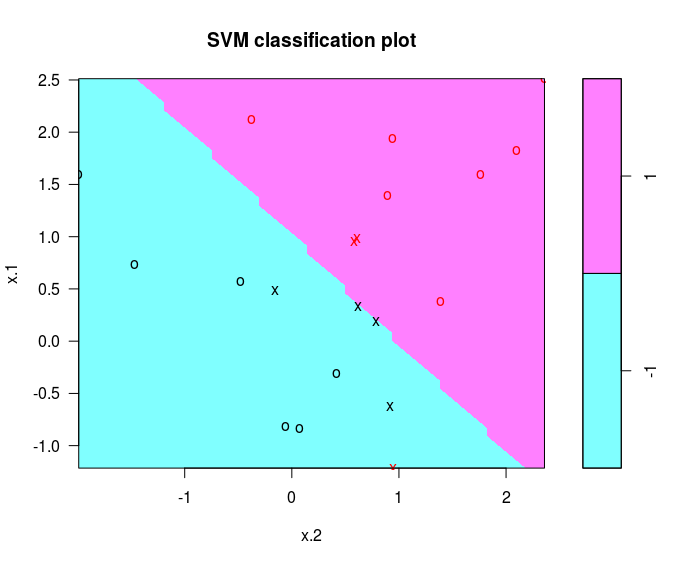svmfit$index summary(svmfit)  ### a smaller cost svmfit = svm ( y ∼. , data = dat, kernel = "linear" , cost = 0.1, scale = FALSE ) plot(svmfit, dat) svmfit$index


After setting a smaller cost, we will get a larger number of support vectors, because the margin is now wider.

### cross-validation

We can use tune()” to perform ten-fold cross-validation on a set of models of interest.

set.seed(1)
tune.out = tune (svm, y ~ ., data = dat, kernel ="linear",
ranges = list ( cost = c (0.001 , 0.01 , 0.1 , 1 ,5 ,10 ,100) ) )


The output is as follows: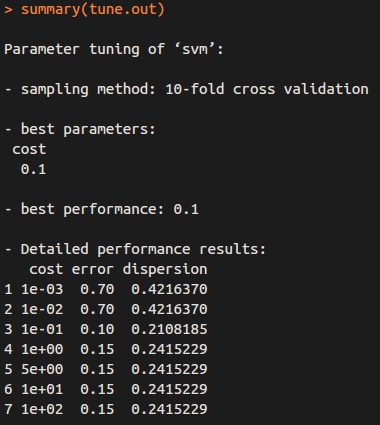So “cost = 0.1” results in the lowest cross-validation error rate, and we can access the best model as follows:

bestmod = tune.out$best.model summary( bestmod )  ### prediction xtest = matrix ( rnorm (20*2) , ncol =2) ytest = sample ( c ( -1 ,1) , 20 , rep = TRUE ) xtest [ ytest ==1 ,]= xtest [ ytest ==1, ] + 1 testdat = data.frame ( x = xtest , y = as.factor ( ytest ) ) ypred = predict ( bestmod , testdat ) table ( predict = ypred , truth = testdat$y )


## Support Vector Machine

### generate some data with a non-linear class boundary

set.seed(1)
x = matrix ( rnorm (200*2) , ncol =2)
x [1:100, ]= x [1:100, ]+2
x [101:150, ]= x [101:150, ] -2
y = c ( rep(1, 150) , rep(2, 50) )
dat = data.frame ( x =x , y = as.factor ( y ) )
plot(x, col = y)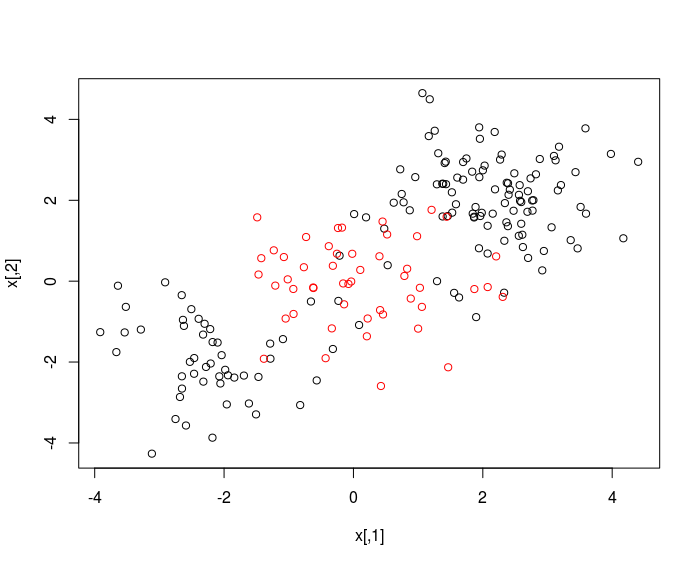### randomly split into training and testing groups.

train = sample (200 ,100)


### training data using radical kernel

svmfit = svm(y~. , data = dat [ train ,], kernel = "radial", cost =1)
plot ( svmfit , dat [ train ,])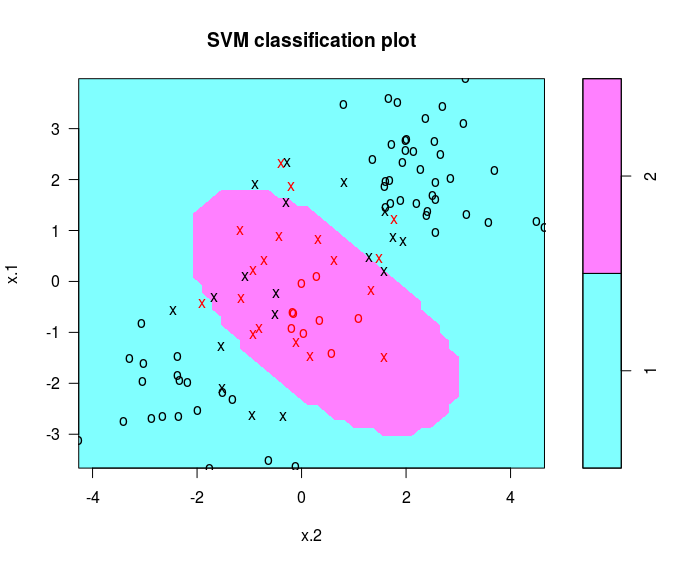### cross-validation

set.seed (1)
tune.out = tune(svm, y∼. , data = dat [ train ,] , kernel =" radial " ,
ranges = list ( cost = c (0.1 ,1 ,10 ,100 ,1000) ,
gamma = c (0.5 ,1 ,2 ,3 ,4) ) )
summary( tune.out )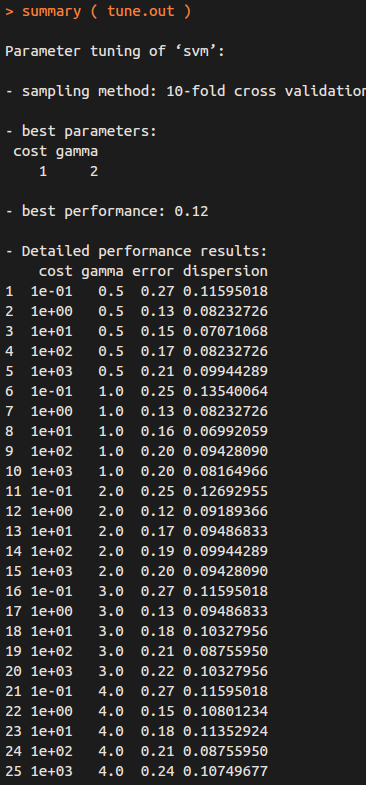Therefore, the best choice of parameters involves “cost=1” and “gamma=2”.

table( true = dat [ - train ,"y"] , pred = predict ( tune.out$best.model, newdata = dat [ - train ,]) )  ## ROC Curves The ROC curve is a popular graphic for simultaneously displaying the two types of errors for all possible thresholds. Varying the classifier threshold changes its true positive rate (sensitivity) and false positive rate (1 - specificity).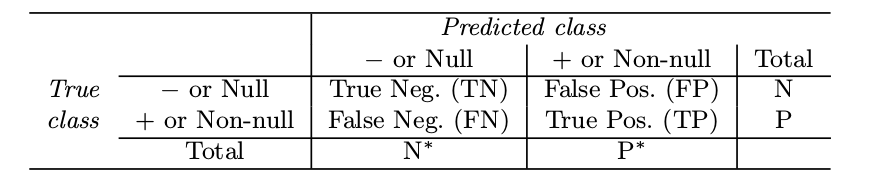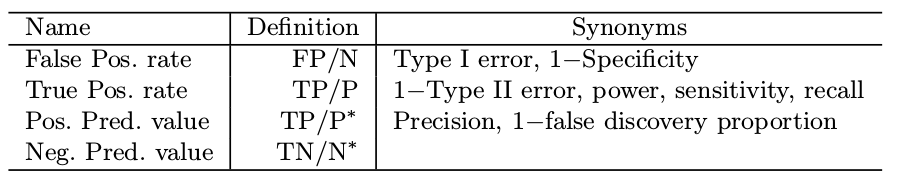The overall performance of a classifier, summarized over all possible thresholds, is given by the area under the (ROC) curve (AUC). An ideal ROC curve will hug the top left corner, so the larger the AUC the better the classifier. The ROCR package can be used to produce ROC curves. library(ROCR) rocplot = function ( pred , truth , ...) { predob = prediction ( pred, truth ) perf = performance ( predob , "tpr" , "fpr") plot ( perf,...) }  svmfit.opt = svm (y~., data = dat[train, ], kernel = "radial", gamma =2 , cost =1 , decision.values = T ) fitted = attributes(predict(svmfit.opt, dat[train, ], decision.values = TRUE))$decision.values

par ( mfrow = c (1 ,2) )
rocplot ( fitted , dat [ train ,"y"] , main ="Training Data")


By increasing $\gamma$ we can produce a more flexible fit and generate further improvements in accuracy.

svmfit.flex = svm(y~. , data = dat [ train ,] , kernel = "radial", gamma =50 , cost =1 , decision.values = T )
fitted = attributes ( predict ( svmfit.flex , dat [ train ,] , decision.values = T ) ) $decision.values rocplot ( fitted , dat [ train ,"y"] , add =T , col ="red")  But on the test data,$\gamma = 2$would be better. fitted = attributes(predict(svmfit.opt, dat[-train, ], decision.values = T ) )$decision.values
rocplot(fitted, dat[-train, "y"] , main = "Test Data")
fitted = attributes(predict(svmfit.flex, dat[-train, ], decision.values = T ) ) $decision.values rocplot(fitted, dat[-train, "y"] , add =T, col ="red")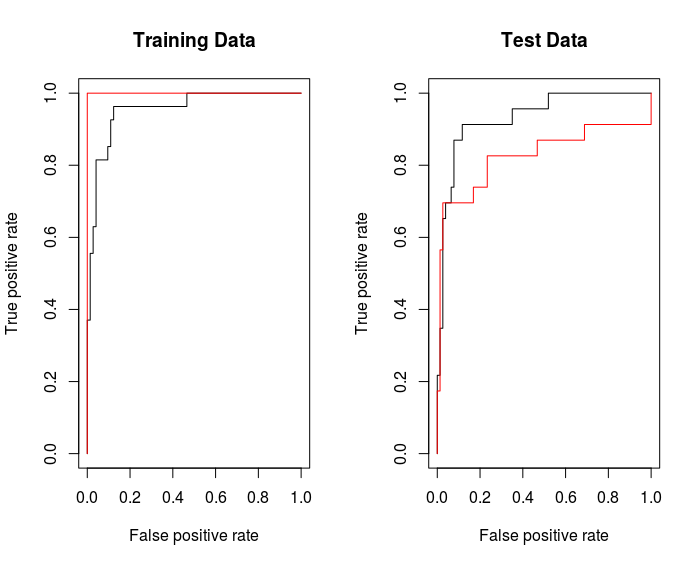## SVM with Multiple Classes set.seed (1) x = rbind (x, matrix ( rnorm (50*2) , ncol =2) ) y = c ( y , rep (0 ,50) ) x [ y ==0 ,2]= x [ y ==0 ,2]+2 dat = data.frame ( x =x , y = as.factor ( y ) ) par(mfrow = c (1 ,1) ) plot (x , col = (y +1) )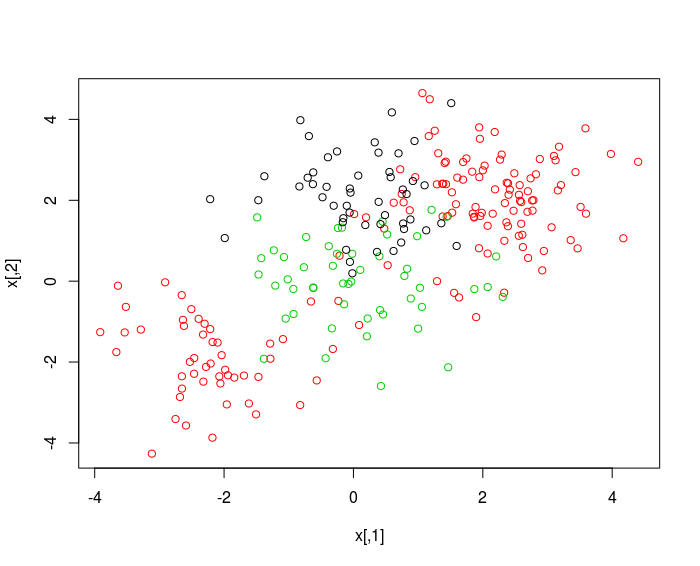svmfit = svm(y~., data = dat , kernel ="radial" , cost =10 , gamma =1) plot ( svmfit , dat )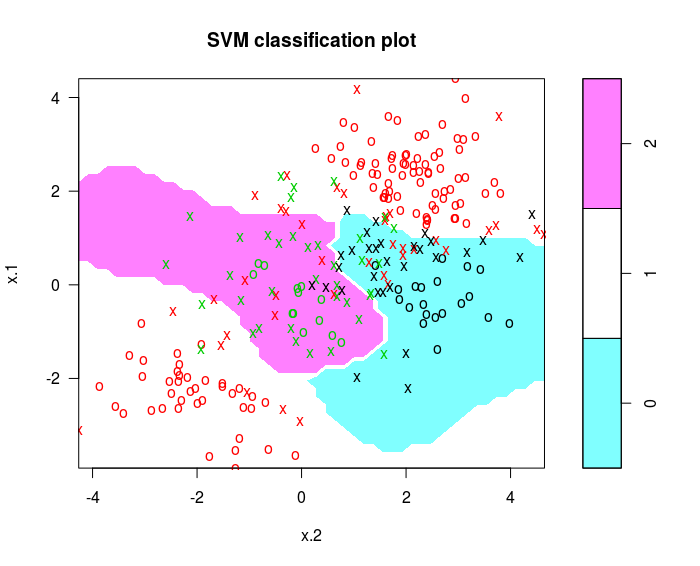The e1071 library can also be used to perform support vector regression, if the response vector that is passed in to svm() is numerical rather than a factor. for classification (y is factor)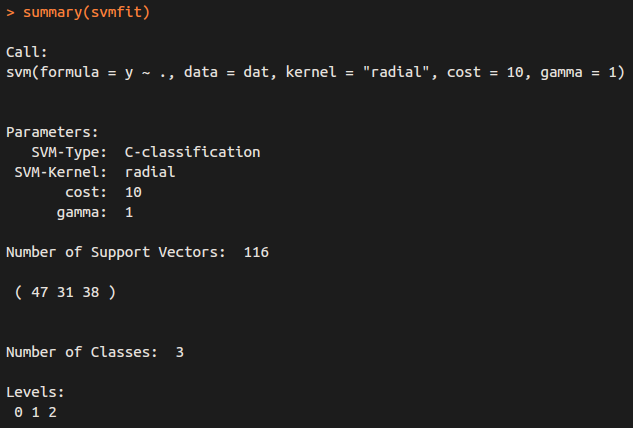for regression (y is NOT factor)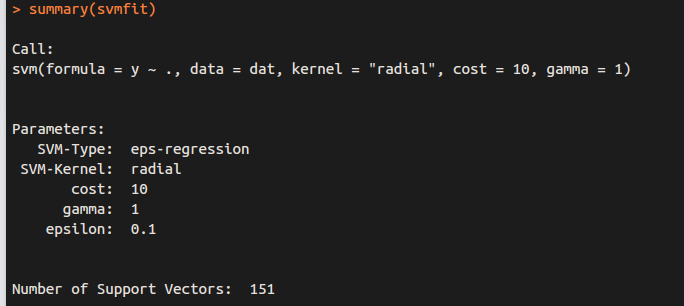## Application to Gene Expression Data ### examine the dimension of the data library(ISLR) names(Khan) dim(Khan$xtrain)
dim(Khan$xtest) length(Khan$ytrain)
length(Khan$ytest) table(Khan$ytrain)
table(Khan$ytest)  dat = data.frame ( x = Khan$xtrain, y = as.factor(Khan$ytrain)) out = svm ( y~., data = dat , kernel = "linear", cost =10) summary(out) table ( out$fitted , dat$y )  NO training errors. In fact, this is not surprising, because the large number of variables relative to the number of observations implies that it is easy to find hyperplanes that fully separate the classes. dat.te = data.frame ( x = Khan$xtest , y = as.factor( Khan$ytest ) ) pred.te = predict ( out, newdata = dat.te ) table ( pred.te , dat.te$y )


## Reference

An Introduction to Statistical Learning with Applications in R

Published in categories Note